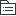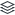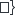# 音乐教育（美育）

32
|
5

## 相关模板推荐

•## 音乐教育（美育） —— 作品大纲

•审美
• 自然美
• 艺术美
• 视觉艺术
• 美术
• 听觉艺术
• 音乐美学原理
• 外部动态（体裁形式）
• 优美
• 壮美
• 内在本质（情感、内容）
• 崇高美
• 欢乐美
• 悲剧美
• 戏剧美
• 音乐
• 概念
• 乐记
• 定义
• 音响
• 情感
• 艺术形式
• 属性
• 物理属性
• 音高
• 音值
• 音强
• 音色
• 社会属性
• 民族性
• 阶级性
• 人民性
• 时代性
• 功能
• 审美功能
• 教育功能
• 认识功能
• 娱乐功能
• 实用功能
• 特征
• 声音的艺术
• 音响
• 时间的艺术
• 记忆
• 听觉的艺术
• 感知、联想、想象
• 情感的艺术
• 表达
• 语言艺术
• 文学
• 综合艺术
• 电影
• 电视
• 戏剧
• 社会美
•中小学音乐教育
• 性质
• 社会科学领域的交叉性学科
• 心理学
• 教育学
• 音乐美学
• 音乐哲学
• 音乐学
•课程性质第一条：人文性
• 对音乐教育的依存
• 属于音乐教育
• 课程性质：审美性
• 鲜明的实践性
• 音乐美的感知、鉴赏、理解和表现必须通过亲身感受、体验才能实现
• 课程性质：实践性
• 任务
• “塑人”
• 培养学生具有正确的音乐审美观
• 态度
• 兴趣
• 体验
• 培养学生对音乐美的感受能力
• 用音乐教育人，教会人用音乐
• 面向全体学生，注重个性发展
• 培养学生对音乐美的鉴赏能力
• 培养学生对音乐美的表现能力
• 培养学生对音乐美的创造能力
• 总目标
• 通过音乐课程学习和参与丰富多样的艺术时间活动
• 培养良好的审美情趣和积极乐观的生活态度
• 培养对音乐的持久兴趣，涵养美感，和谐身心，陶冶情操，健全人格
• 掌握必要的双基
• 发展音乐听觉与欣赏能力、表现能力和创造能力形成基本的音乐素养
• 特征
• 音乐艺术的审美特性
• 音乐是抽象的而非语义性的
• 音乐是表情性的而非摹拟性的
• 音乐是创造性的而非同一性的
• 以音乐审美为核心
• 音乐是传情性的而非具象性的
• 音乐是时空性的而非静态的
• 音乐教育的特征
• 情感性
• 情感唤起阶段
• 以爱美为特征激发培养学生对音乐的学习兴趣
• 情感体验深入阶段
• 以鉴赏美为特征，培养学生感受、体验、鉴赏、评价音乐美
• 情感外化阶段
• 以创造美为特征，将内在审美情感体验客观化、对象化、形式化
• 形象性
• 通过复杂的人类艺术形象思维一一联想、幻想、想象等一系列的阶梯，从而间接表现出客观世界
• 愉悦性
• 自身愉悦感
• 教学方式
• 过程的愉悦
• 要以兴趣爱好为动力
• 主体性
• 创作主体
• 表演主体
• 强调音乐实践，鼓励音乐创造
• 欣赏主体
• 价值取向
• 审美体验价值
• 教学中，应充分揭示音乐要素（节奏、旋律、音色、和声、力度、速度等）在音乐中的表现作用，让学生亲身感受到音乐中最激动人心和极具表现力的部分，而不是进行枯燥的、单纯的技巧训练和灌输死记硬背的知识；应以整体的方式来体验音乐，理解“整体大于元素的总和”这一心理学原理，使学生感受到音乐的魅力，产生情绪的反映和情感的体验，而不是将音乐的各种构成元素支离破碎地讲授。
• 这是音乐教育区别其他学科的一项重要的原则
• 创造性发展价值
• 音乐的产生过程就是全部的创作过程
• 音乐具有“不确定性”特点
• 音乐的情感特征可以极大地激发学生的表现欲望和创造热情
• 表现与社会交往价值
• 音乐教育为学生提供了创造性表达个人情感的可能性
• 文化传承价值
• 传承传统音乐文化是音乐教育的主要任务之一
• 功能
• 主体效应
• 是学校实施美育的主要内容和途径
• 是学校实施美育的最佳方式
• 是学校实施美育的有效手段
• 协同效应
• 辅德
• 益智
• 心理功能
• 感知
• 记忆
• 注意
• 情感
• 联想
• 想象
• 兴趣
• 意志
• 理解
• 健体
• 音乐教育能促进体育的发展，对学生身心健康协调发展起积极作用
• 文化效应
• 具有传递音乐文化的作用
• 具有选择音乐文化的作用
• 具有整理音乐文化的作用
• 具有发展音乐文化的作用
•弘扬民族音乐，理解音乐文化多样性
• 原则
• 原则依据
• 音乐教学实践的经验
• 音乐教育教学目的
• 音乐自身教学规律
• 现代教学理论基础
• 教学基本原则
• 音乐本位原则
• ---体现音乐教学的方向
• 协同融合原则
• ---体现音乐教学发展趋势
• 创造发展原则
• ---体现音乐教学的旨归
• 情感体验原则
• ---体现音乐教学的主过程
• 主动参与原则
• ---体现音乐教学主要方式手段
•音乐教育实践性的体现与分化
•音乐教学中带根本性规律的反映
• 内容划分
• 课程结构
• 感受与欣赏
• 音乐表现要素
• 音乐情绪与情感
• 音乐体裁与形式
• 音乐风格与流派
• 表现
• 演唱
• 演奏
• 综合性要素表演
• 识读乐谱
• 创造
• 探索音响与音乐
• 即兴创编
• 创作实践
• 音乐与相关文化
• 音乐与社会生活
• 音乐与姊妹艺术
• 音乐与艺术之外的其他学科
• 课堂教学
• 单一课
• 唱歌课
• 定位
• 内容要求
• 特点分析
• 流程步骤
• 认知--记忆--理解（听辨）--运用
• 教学原则
• 教学方法
• 评价
• 方式
• 标准
• 器乐课
• 欣赏课
• 综合课
• 戏剧课
• 创作课
• 唱游课
• 教育目标分类
• 要素型学习目标（短期达成）
• 显性
• 陈述性知识（知识）
• 掌握音乐基本要素
• 力度、速度、音色、节奏、节拍、旋律、和声、调式等
• 常见结构
• 歌曲
• 通节歌
• 分节歌
• 乐曲
• 一部曲式
• 二部曲式
• 单三曲式
• 复三曲式
• 体裁形式
• 歌曲
• 民歌
• 山歌
• 小调
• 劳动号子
• 民歌改编
• 艺术歌曲
• 国内艺术歌曲
• 国外艺术歌曲
• 群众歌曲
• 抗战歌曲
• 音乐剧
• 音乐喜剧
• 音乐舞剧
• 影视歌曲
• 主题歌
• 插曲
• 歌剧
• 轻歌剧
• 正歌剧
• 器乐曲
• 标题音乐与无标题音乐
• 无标题音乐
• 奏鸣曲
• 小步舞曲
• 标题音乐
• 以文字或标题阐明作品思想内容的器乐作品
• 室内乐和交响音乐
• 室内乐
• 交响音乐
• 单声音乐和多声音乐
• 主调音乐
• 复调音乐
• 歌剧音乐
• 序曲
• 咏叹调
• 宣叙调
• 风格流派
• 风格
• 古典音乐
• 流行音乐
• 民族民间音乐
• 世界音乐
• 流派
• 古典乐派（18世纪初到十九世纪二十年代）
• 浪漫乐派（19世纪）
• 民族乐派（19世纪中期）
• 现代乐派（19世纪末）
• 印象主义（20世纪）
• 唱、奏、识谱、编创等基础知识
• 程序性知识（技能）
• 演唱
• 歌唱姿势
• 呼吸方法
• 咬字吐字
• 发声技巧
• 情感表达
• 演奏
• 演奏姿势
• 演奏技巧
• 识读乐谱
• 乐曲表现
• 创作
• 图式
• 隐性
• 表象
• 前概念
• 狭义的经验
• 思维
• 思维方法
• 思维产品
• 思维品质
• 元认知策略
• 体验型学习目标（养成）
• 情感
• 态度
• 价值观
• 融合型学习目标（长期养成）
• 观念
• 行为
• 习惯
• 能力
• 品格
• 智慧
• 素养
• 相关教学法
• 雅克·达尔克罗兹
• 教育思想
• 音乐使人生活的更美好
• 教学理念
• 音乐是律动的艺术，用身体感受音乐的运动，产生律动，表达情感
• 教学目标
• 用体态律动把乐曲情感化为可感可听可见的节奏动作与声音，以唤起体验本能
• 教学内容
• 通过体态律动、视唱练耳和即兴创作获得基本的音乐活动能力
• 教学方法
• 即兴式律动反应，用体态律动感受、体验、表现、表达音乐
• 卡尔·奥尔夫
• 教育思想
• 每个人都能学会和体验音乐
• 教学理念
• 诉诸感性，以人为本，唤醒、开发和提升人表达情感的音乐本能
• 教学目标
• 让学生在群体即兴、游戏中愉悦身心，学习和体验音乐
• 教学内容
• 音乐-语言-动作三结合的音乐活动、奥尔夫乐器演奏
• 教学方法
• 原本性、元素性、即兴创造。强调综合性、即兴性和参与性
• 佐尔坦·柯达伊
• 教育思想
• 让音乐属于每一个人
• 教学理念
• 民间音乐与歌唱是音乐教育的双重基础
• 教学目标
• 培养有音乐修养的音乐家和音乐爱好者
• 教学内容
• 从民歌到经典合唱的系统性歌唱教程
• 教学方法
• 以歌唱作为一切音乐教学的出发点和依据
• 音乐教育新体系
• 教育思想
• 面向全体学生，音乐教育让人更美好
• 教学理念
• 融以情感人，以美动人，立德树人为一体，让学生在教学中充分体验音乐中的真善美
• 教学目标
• 使学生获得感性音乐经验，作为爱好音乐、学音乐的基础用音乐
• 教学内容
• 以综合性音乐情感体验实践活动为教学主线和主要教学内容
• 教学方法
• 体验式教学法：以听、唱、动、视、创等联感联觉培养感知、表达、表现、想象、创造、交流与合作等音乐活动能力
•教育
• 音乐教育历史
• 古代的音乐教育
• 近现代的音乐教育
• 西方的音乐教育
• 心理学
• 理论观点
• 行为主义
• 认知主义
• 儿童发展
• 皮亚杰阶段理论
• 其他观点
• 对音乐教学的启示
• 认知过程
• 两类过程
• 人类信息加工
• 动机
• 竞争、成就动机
• 自我动机
• 人本主义动机
• 环境因素
• 音乐能力
• 教育学
• 教育目标分类
• 行为化表述
• 戈登学习理论
• 教学法
• 音乐哲学
• 三种基本观点
• 唯理主义
• 经验主义
• 实用主义
• 三种优缺点
• 哲学观点与教育
• 社会学
• 自我意象
• 从众
• 角色和期待
• 竞争与合作
• 领导能力
• 自信感
• 态度
• 创造力